# Time Series Machine Learning

#### 2020-05-30

A collection of tools for working with time series in R

The time series signature is a collection of useful features that describe the time series index of a time-based data set. It contains a wealth of features that can be used to forecast time series that contain patterns. In this vignette, the user will learn methods to implement machine learning to predict future outcomes in a time-based data set. The vignette example uses a well known time series dataset, the Bike Sharing Dataset, from the UCI Machine Learning Repository. The vignette follows an example where we’ll use `timetk` to build a basic Machine Learning model to predict future values using the time series signature. The objective is to build a model and predict the next six months of Bike Sharing daily counts.

# Prerequisites

Before we get started, load the following packages.

``````library(workflows)
library(parsnip)
library(recipes)
library(yardstick)
library(tidyverse)
library(tidyquant)
library(timetk)``````

# Data

We’ll be using the Bike Sharing Dataset from the UCI Machine Learning Repository.

Source: Fanaee-T, Hadi, and Gama, Joao, ‘Event labeling combining ensemble detectors and background knowledge’, Progress in Artificial Intelligence (2013): pp. 1-15, Springer Berlin Heidelberg

``````# Read data
bikes_tbl <- bike_sharing_daily %>%
select(dteday, cnt) %>%
rename(date  = dteday,
value = cnt)

bikes_tbl``````
``````## # A tibble: 731 x 2
##    date       value
##    <date>     <dbl>
##  1 2011-01-01   985
##  2 2011-01-02   801
##  3 2011-01-03  1349
##  4 2011-01-04  1562
##  5 2011-01-05  1600
##  6 2011-01-06  1606
##  7 2011-01-07  1510
##  8 2011-01-08   959
##  9 2011-01-09   822
## 10 2011-01-10  1321
## # … with 721 more rows``````

A visualization will help understand how we plan to tackle the problem of forecasting the data. We’ll split the data into two regions: a training region and a testing region.

``````# Visualize data and training/testing regions
bikes_tbl %>%
ggplot(aes(x = date, y = value)) +
geom_rect(xmin = as.numeric(ymd("2012-07-01")),
xmax = as.numeric(ymd("2013-01-01")),
ymin = 0, ymax = 10000,
fill = palette_light()[], alpha = 0.01) +
annotate("text", x = ymd("2011-10-01"), y = 7800,
color = palette_light()[], label = "Train Region") +
annotate("text", x = ymd("2012-10-01"), y = 1550,
color = palette_light()[], label = "Test Region") +
geom_point(alpha = 0.5, color = palette_light()[]) +
labs(title = "Bikes Sharing Dataset: Daily Scale", x = "") +
theme_tq()``````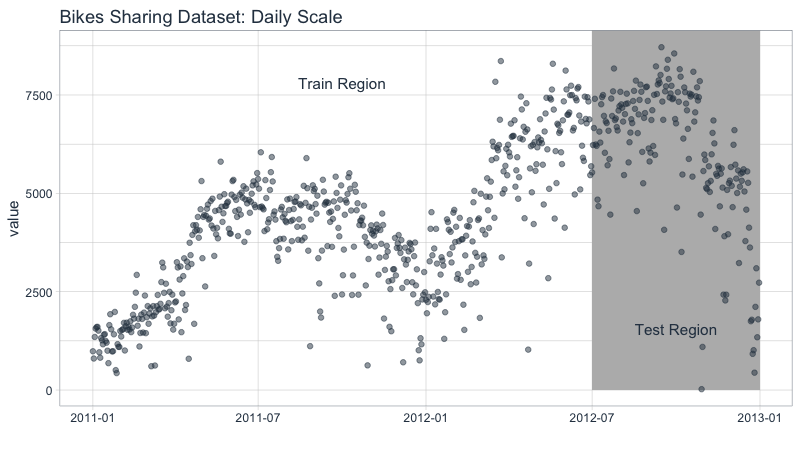Split the data into train and test sets at “2012-07-01”.

``````# Split into training and test sets
train_tbl <- bikes_tbl %>% filter(date < ymd("2012-07-01"))
test_tbl  <- bikes_tbl %>% filter(date >= ymd("2012-07-01"))``````

# Modeling

Start with the training set, which has the “date” and “value” columns.

``````# Training set
train_tbl``````
``````## # A tibble: 547 x 2
##    date       value
##    <date>     <dbl>
##  1 2011-01-01   985
##  2 2011-01-02   801
##  3 2011-01-03  1349
##  4 2011-01-04  1562
##  5 2011-01-05  1600
##  6 2011-01-06  1606
##  7 2011-01-07  1510
##  8 2011-01-08   959
##  9 2011-01-09   822
## 10 2011-01-10  1321
## # … with 537 more rows``````

## Recipe Preprocessing Specification

The first step is to add the time series signature to the training set, which will be used this to learn the patterns. New in `timetk` 0.1.3 is integration with the `recipes` R package:

• The `recipes` package allows us to add preprocessing steps that are applied sequentially as part of a data transformation pipeline.

• The `timetk` has `step_timeseries_signature()`, which is used to add a number of features that can help machine learning models.

``````# Add time series signature
recipe_spec_timeseries <- recipe(value ~ ., data = train_tbl) %>%
step_timeseries_signature(date) ``````

We can see what happens when we apply a prepared recipe `prep()` using the `bake()` function. Many new columns were added from the timestamp “date” feature. These are features we can use in our machine learning models.

``bake(prep(recipe_spec_timeseries), new_data = train_tbl)``
``````## # A tibble: 547 x 29
##    date       value date_index.num date_year date_year.iso date_half
##    <date>     <dbl>          <int>     <int>         <int>     <int>
##  1 2011-01-01   985     1293840000      2011          2010         1
##  2 2011-01-02   801     1293926400      2011          2010         1
##  3 2011-01-03  1349     1294012800      2011          2011         1
##  4 2011-01-04  1562     1294099200      2011          2011         1
##  5 2011-01-05  1600     1294185600      2011          2011         1
##  6 2011-01-06  1606     1294272000      2011          2011         1
##  7 2011-01-07  1510     1294358400      2011          2011         1
##  8 2011-01-08   959     1294444800      2011          2011         1
##  9 2011-01-09   822     1294531200      2011          2011         1
## 10 2011-01-10  1321     1294617600      2011          2011         1
## # … with 537 more rows, and 23 more variables: date_quarter <int>,
## #   date_month <int>, date_month.xts <int>, date_month.lbl <ord>,
## #   date_day <int>, date_hour <int>, date_minute <int>, date_second <int>,
## #   date_hour12 <int>, date_am.pm <int>, date_wday <int>, date_wday.xts <int>,
## #   date_wday.lbl <ord>, date_mday <int>, date_qday <int>, date_yday <int>,
## #   date_mweek <int>, date_week <int>, date_week.iso <int>, date_week2 <int>,
## #   date_week3 <int>, date_week4 <int>, date_mday7 <int>``````

Next, I apply various preprocessing steps to improve the modeling behavior. If you wish to learn more, I have an Advanced Time Series course that will help you learn these techniques.

``````recipe_spec_final <- recipe_spec_timeseries %>%
step_rm(date) %>%
step_rm(contains("iso"), contains("minute"), contains("hour"),
contains("am.pm"), contains("xts")) %>%
step_normalize(contains("index.num"), date_year) %>%
step_dummy(contains("lbl"), one_hot = TRUE)

bake(prep(recipe_spec_final), new_data = train_tbl)``````
``````## # A tibble: 547 x 37
##    value date_index.num date_year date_half date_quarter date_month date_day
##    <dbl>          <dbl>     <dbl>     <int>        <int>      <int>    <int>
##  1   985          -1.73    -0.705         1            1          1        1
##  2   801          -1.72    -0.705         1            1          1        2
##  3  1349          -1.71    -0.705         1            1          1        3
##  4  1562          -1.71    -0.705         1            1          1        4
##  5  1600          -1.70    -0.705         1            1          1        5
##  6  1606          -1.70    -0.705         1            1          1        6
##  7  1510          -1.69    -0.705         1            1          1        7
##  8   959          -1.68    -0.705         1            1          1        8
##  9   822          -1.68    -0.705         1            1          1        9
## 10  1321          -1.67    -0.705         1            1          1       10
## # … with 537 more rows, and 30 more variables: date_second <int>,
## #   date_wday <int>, date_mday <int>, date_qday <int>, date_yday <int>,
## #   date_mweek <int>, date_week <int>, date_week2 <int>, date_week3 <int>,
## #   date_week4 <int>, date_mday7 <int>, date_month.lbl_01 <dbl>,
## #   date_month.lbl_02 <dbl>, date_month.lbl_03 <dbl>, date_month.lbl_04 <dbl>,
## #   date_month.lbl_05 <dbl>, date_month.lbl_06 <dbl>, date_month.lbl_07 <dbl>,
## #   date_month.lbl_08 <dbl>, date_month.lbl_09 <dbl>, date_month.lbl_10 <dbl>,
## #   date_month.lbl_11 <dbl>, date_month.lbl_12 <dbl>, date_wday.lbl_1 <dbl>,
## #   date_wday.lbl_2 <dbl>, date_wday.lbl_3 <dbl>, date_wday.lbl_4 <dbl>,
## #   date_wday.lbl_5 <dbl>, date_wday.lbl_6 <dbl>, date_wday.lbl_7 <dbl>``````

## Model Specification

Next, let’s create a model specification. We’ll use a `glmnet`.

``````model_spec_glmnet <- linear_reg(mode = "regression") %>%
set_engine("lm")``````

## Workflow

We can mary up the preprocessing recipe and the model using a `workflow()`.

``````workflow_glmnet <- workflow() %>%

workflow_glmnet``````
``````## ══ Workflow ═════════════════════════════════════════════════════════════════════════════════════════════════════════
## Preprocessor: Recipe
## Model: linear_reg()
##
## ── Preprocessor ─────────────────────────────────────────────────────────────────────────────────────────────────────
## 5 Recipe Steps
##
## ● step_timeseries_signature()
## ● step_rm()
## ● step_rm()
## ● step_normalize()
## ● step_dummy()
##
## ── Model ────────────────────────────────────────────────────────────────────────────────────────────────────────────
## Linear Regression Model Specification (regression)
##
## Computational engine: lm``````

## Training

The workflow can be trained with the `fit()` function.

``workflow_trained <- workflow_glmnet %>% fit(data = train_tbl)``

## Test (Validation)

With a suitable model in hand, we can forecast using the “test” set for validation purposes.

``````prediction_tbl <- workflow_trained %>%
predict(test_tbl) %>%
bind_cols(test_tbl)

prediction_tbl``````
``````## # A tibble: 184 x 3
##    .pred date       value
##    <dbl> <date>     <dbl>
##  1 6237. 2012-07-01  5531
##  2 6641. 2012-07-02  6227
##  3 6850. 2012-07-03  6660
##  4 6691. 2012-07-04  7403
##  5 6919. 2012-07-05  6241
##  6 6941. 2012-07-06  6207
##  7 6956. 2012-07-07  4840
##  8 6617. 2012-07-08  4672
##  9 6624. 2012-07-09  6569
## 10 6833. 2012-07-10  6290
## # … with 174 more rows``````

Visualize the results using `ggplot()`.

``````ggplot(aes(x = date), data = bikes_tbl) +
geom_rect(xmin = as.numeric(ymd("2012-07-01")),
xmax = as.numeric(ymd("2013-01-01")),
ymin = 0, ymax = 10000,
fill = palette_light()[], alpha = 0.01) +
annotate("text", x = ymd("2011-10-01"), y = 7800,
color = palette_light()[], label = "Train Region") +
annotate("text", x = ymd("2012-10-01"), y = 1550,
color = palette_light()[], label = "Test Region") +
geom_point(aes(x = date, y = value),
alpha = 0.5, color = palette_light()[]) +
geom_point(aes(x = date, y = .pred), data = prediction_tbl,
alpha = 0.5, color = palette_light()[]) +
theme_tq() ``````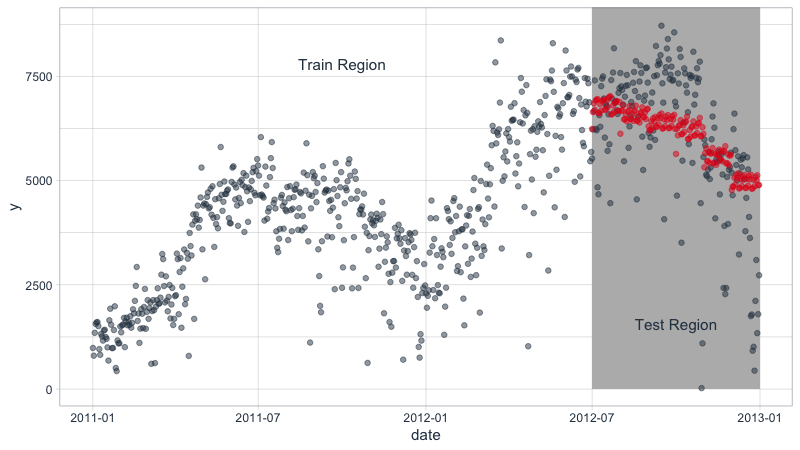## Validation Accuracy (Out of Sample)

The Out-of-Sample Forecast Accuracy can be measured with `yardstick`.

``````# Calculating forecast error
prediction_tbl %>% metrics(value, .pred)``````
``````## # A tibble: 3 x 3
##   .metric .estimator .estimate
##   <chr>   <chr>          <dbl>
## 1 rmse    standard    1437.
## 2 rsq     standard       0.379
## 3 mae     standard    1045.``````

Next we can visualize the residuals of the test set. The residuals of the model aren’t perfect, but we can work with it. The residuals show that the model predicts low in October and high in December.

``````prediction_tbl %>%
ggplot(aes(x = date, y = value - .pred)) +
geom_hline(yintercept = 0, color = "red") +
geom_point(color = palette_light()[], alpha = 0.5) +
geom_smooth() +
theme_tq() +
labs(title = "Test Set: GLM Model Residuals", x = "") +
scale_y_continuous(limits = c(-5000, 5000))``````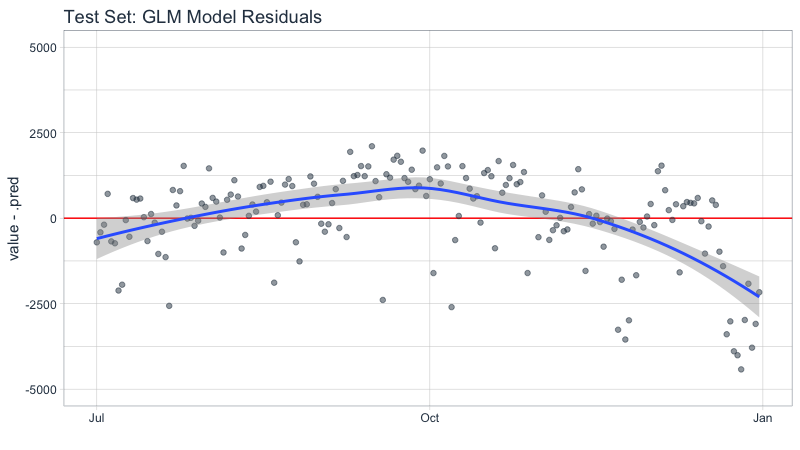This is certainly not the best model we can come up with, but it will do for a quick tutorial. I have a ton of improvements that can be made, and I teach these expert techniques. If you are interested in learning from my advanced Time Series Analysis & Forecasting Course, then join my waitlist. The course is coming soon.

# Forecasting Future Data

Let’s use our model to predict What are the expected future values for the next six months. The first step is to create the date sequence. Let’s use `tk_get_timeseries_summary()` to review the summary of the dates from the original dataset, “bikes”.

``````# Extract bikes index
idx <- bikes_tbl %>% tk_index()

# Get time series summary from index
bikes_summary <- idx %>% tk_get_timeseries_summary()``````

The first six parameters are general summary information.

``bikes_summary[1:6]``
``````## # A tibble: 1 x 6
##   n.obs start      end        units scale tzone
##   <int> <date>     <date>     <chr> <chr> <chr>
## 1   731 2011-01-01 2012-12-31 days  day   UTC``````

The second six parameters are the periodicity information.

``bikes_summary[7:12]``
``````## # A tibble: 1 x 6
##   diff.minimum diff.q1 diff.median diff.mean diff.q3 diff.maximum
##          <dbl>   <dbl>       <dbl>     <dbl>   <dbl>        <dbl>
## 1        86400   86400       86400     86400   86400        86400``````

From the summary, we know that the data is 100% regular because the median and mean differences are 86400 seconds or 1 day. We don’t need to do any special inspections when we use `tk_make_future_timeseries()`. If the data was irregular, meaning weekends or holidays were excluded, you’d want to account for this. Otherwise your forecast would be inaccurate.

``````idx_future <- idx %>% tk_make_future_timeseries(length_out = 180)

future_tbl <- tibble(date = idx_future)

future_tbl``````
``````## # A tibble: 180 x 1
##    date
##    <date>
##  1 2013-01-01
##  2 2013-01-02
##  3 2013-01-03
##  4 2013-01-04
##  5 2013-01-05
##  6 2013-01-06
##  7 2013-01-07
##  8 2013-01-08
##  9 2013-01-09
## 10 2013-01-10
## # … with 170 more rows``````

Retrain the model specification on the full data set, then predict the next 6-months.

``````future_predictions_tbl <- workflow_glmnet %>%
fit(data = bikes_tbl) %>%
predict(future_tbl) %>%
bind_cols(future_tbl)``````

Visualize the forecast.

``````bikes_tbl %>%
ggplot(aes(x = date, y = value)) +
geom_rect(xmin = as.numeric(ymd("2012-07-01")),
xmax = as.numeric(ymd("2013-01-01")),
ymin = 0, ymax = 10000,
fill = palette_light()[], alpha = 0.01) +
geom_rect(xmin = as.numeric(ymd("2013-01-01")),
xmax = as.numeric(ymd("2013-07-01")),
ymin = 0, ymax = 10000,
fill = palette_light()[], alpha = 0.01) +
annotate("text", x = ymd("2011-10-01"), y = 7800,
color = palette_light()[], label = "Train Region") +
annotate("text", x = ymd("2012-10-01"), y = 1550,
color = palette_light()[], label = "Test Region") +
annotate("text", x = ymd("2013-4-01"), y = 1550,
color = palette_light()[], label = "Forecast Region") +
geom_point(alpha = 0.5, color = palette_light()[]) +
# future data
geom_point(aes(x = date, y = .pred), data = future_predictions_tbl,
alpha = 0.5, color = palette_light()[]) +
geom_smooth(aes(x = date, y = .pred), data = future_predictions_tbl,
method = 'loess') +
labs(title = "Bikes Sharing Dataset: 6-Month Forecast", x = "") +
theme_tq()``````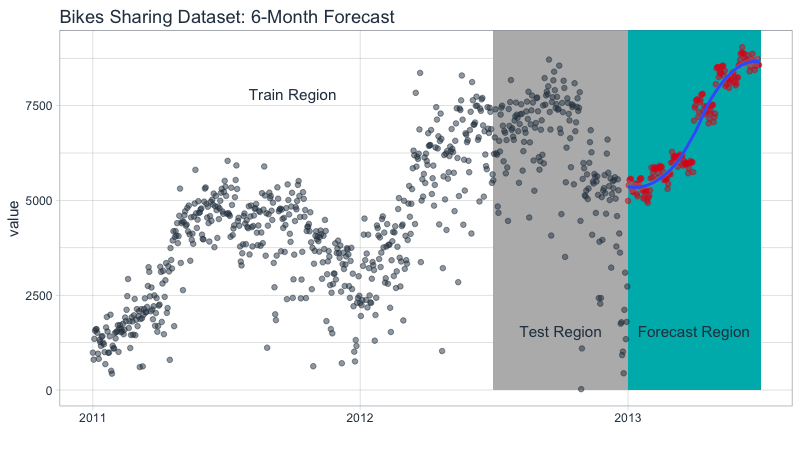# Forecast Error

A forecast is never perfect. We need prediction intervals to account for the variance from the model predictions to the actual data. There’s a number of methods to achieve this. We’ll follow the prediction interval methodology from Forecasting: Principles and Practice.

``````# Calculate standard deviation of residuals
test_resid_sd <- prediction_tbl %>%
summarize(stdev = sd(value - .pred))

future_predictions_tbl <- future_predictions_tbl %>%
mutate(
lo.95 = .pred - 1.96 * test_resid_sd\$stdev,
lo.80 = .pred - 1.28 * test_resid_sd\$stdev,
hi.80 = .pred + 1.28 * test_resid_sd\$stdev,
hi.95 = .pred + 1.96 * test_resid_sd\$stdev
)``````

Now, plotting the forecast with the prediction intervals.

``````bikes_tbl %>%
ggplot(aes(x = date, y = value)) +
geom_point(alpha = 0.5, color = palette_light()[]) +
geom_ribbon(aes(y = .pred, ymin = lo.95, ymax = hi.95),
data = future_predictions_tbl,
fill = "#D5DBFF", color = NA, size = 0) +
geom_ribbon(aes(y = .pred, ymin = lo.80, ymax = hi.80, fill = key),
data = future_predictions_tbl,
fill = "#596DD5", color = NA, size = 0, alpha = 0.8) +
geom_point(aes(x = date, y = .pred), data = future_predictions_tbl,
alpha = 0.5, color = palette_light()[]) +
geom_smooth(aes(x = date, y = .pred), data = future_predictions_tbl,
method = 'loess', color = "white") +
labs(title = "Bikes Sharing Dataset: 6-Month Forecast with Prediction Intervals", x = "") +
theme_tq()``````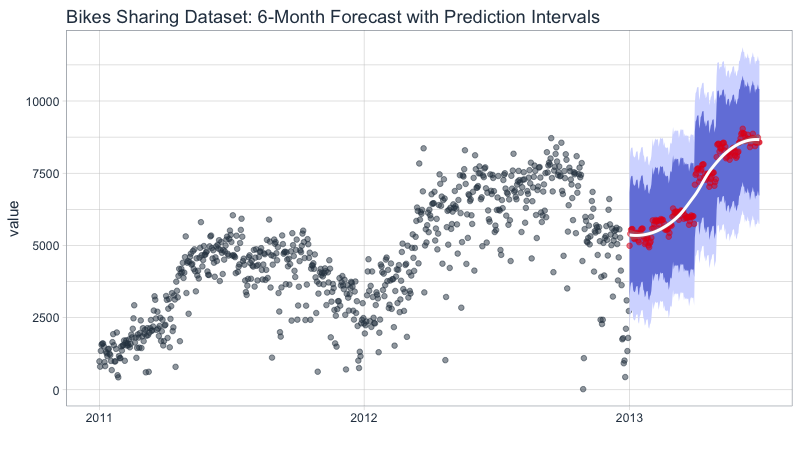# Learning More

If you are interested in learning from my advanced Time Series Analysis & Forecasting Course, then join my waitlist. The course is coming soon.